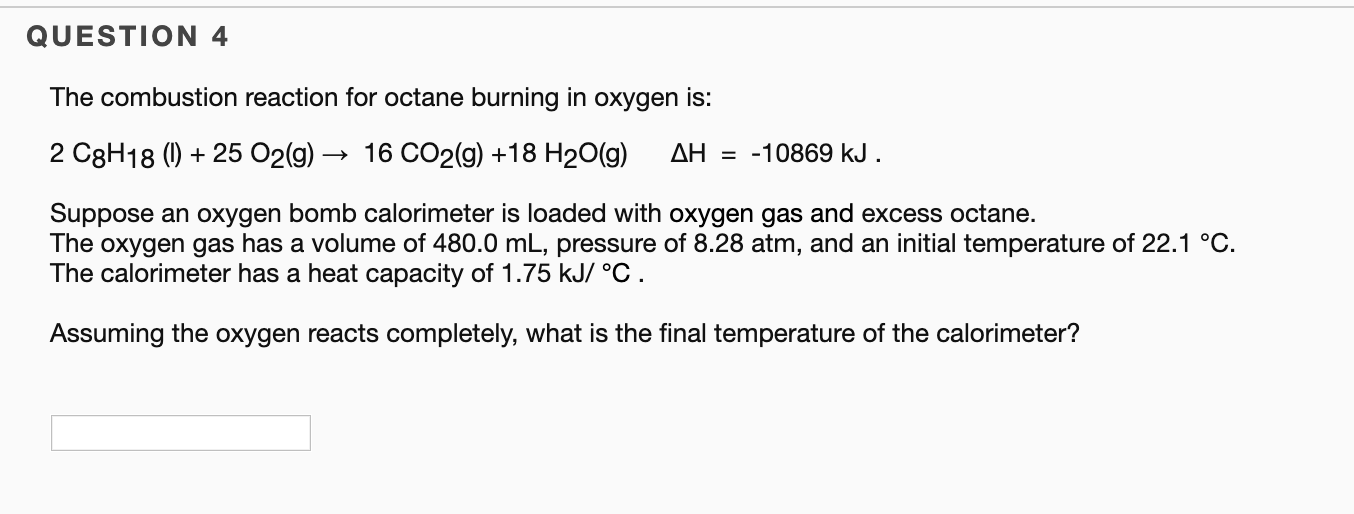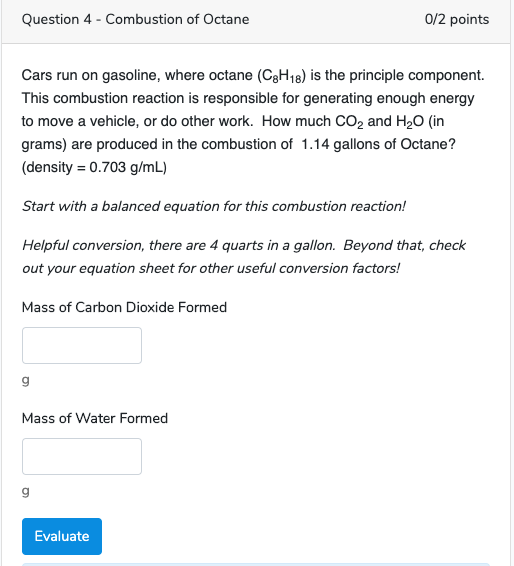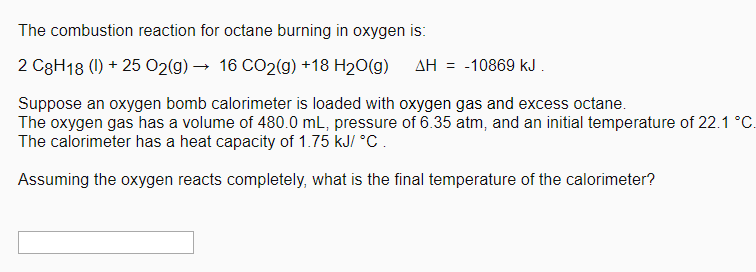Question

# Octane, a component of gasoline, can undergo the following combustion with an excess of oxygen gas:...

Octane, a component of gasoline, can undergo the following combustion with an excess of oxygen gas:
2 C8H18 (ℓ) + 25 O2 (g) → 16 CO2 (g) + 18 H2O (ℓ)

10.0 mL of octane and 1.50 atm of oxygen gas are placed in a 20.0 L container at 20.0°C.

Calculate the total pressure in the container after complete combustion (in atm). (Assume the vapor pressures of water and octane are negligible and temperature and volume remain constant. The density of octane is 0.703 g/mL. The molar mass of octane is 114.22 g/mol.)

We need at least 10 more requests to produce the answer.

0 / 10 have requested this problem solution

The more requests, the faster the answer.

All students who have requested the answer will be notified once they are available.

#### Earn Coins

Coins can be redeemed for fabulous gifts.

Similar Homework Help Questions
• ### The combustion reaction for octane burning in oxygen is: 2 C8H18 (l) + 25 O2(g) →...

The combustion reaction for octane burning in oxygen is: 2 C8H18 (l) + 25 O2(g) → 16 CO2(g) +18 H2O(g) ∆H = -10869 kJ . Suppose an oxygen bomb calorimeter is loaded with oxygen gas and excess octane. The oxygen gas has a volume of 480.0 mL, pressure of 5.67 atm, and an initial temperature of 22.1 °C. The calorimeter has a heat capacity of 1.75 kJ/ °C . Assuming the oxygen reacts completely, what is the final temperature of...

• ### A major component of gasoline is octane (C8H18) . When liquid octane is burned in air it reacts with oxygen (O2) gas to produce carbon dioxide gas and water vapor. Calculate the moles of carbon dioxide produced by the reaction of 1.40 mol of octane. Be su

A major component of gasoline is octane (C8H18) . When liquid octane is burned in air it reacts with oxygen (O2) gas to produce carbon dioxide gas and water vapor. Calculate the moles of carbon dioxide produced by the reaction of 1.40 mol of octane. Be sure your answer has a unit symbol, if necessary, and round it to 3 significant digits.

• ### The complete combustion of octane, C8H18, a component of gasoline, proceeds as follows: 2C8H18(l)+25O2(g)→16CO2(g)+18H2O...

The complete combustion of octane, C8H18, a component of gasoline, proceeds as follows: 2C8H18(l)+25O2(g)→16CO2(g)+18H2O(g) a. How many moles of O2 are needed to burn 1.35 mol of C8H18? b. How many grams of O2 are needed to burn 13.0 g of C8H18? c. Octane has a density of 0.692 g/mL at 20 degrees C. How many grams of O2 are required to burn 1.20 gal of C8H18?

• ### A major component of gasoline is octane C8H18. When octane is burned in air, it chemically...

A major component of gasoline is octane C8H18. When octane is burned in air, it chemically reacts with oxygen gas O2 to produce carbon dioxide CO2 and water H2O. What mass of carbon dioxide is produced by the reaction of 6.46g of octane? Be sure your answer has the correct number of significant digits.

• ### QUESTION 4 The combustion reaction for octane burning in oxygen is: 2 C8H18 ()25 O2(g)- 16...QUESTION 4 The combustion reaction for octane burning in oxygen is: 2 C8H18 ()25 O2(g)- 16 CO2(g) +18 H20(g) AH = -10869 kJ Suppose an oxygen bomb calorimeter is loaded with oxygen gas and excess octane. The oxygen gas has a volume of 480.0 mL, pressure of 8.28 atm, and an initial temperature of 22.1 °C The calorimeter has a heat capacity of 1.75 kJ/ °C Assuming the oxygen reacts completely, what is the final temperature of the calorimeter?

• ### Question 4 - Combustion of Octane 0/2 points Cars run on gasoline, where octane (C8H18) is...Question 4 - Combustion of Octane 0/2 points Cars run on gasoline, where octane (C8H18) is the principle component. This combustion reaction is responsible for generating enough energy to move a vehicle, or do other work. How much co, and H2O (in grams) are produced in the combustion of 1.14 gallons of Octane? (density = 0.703 g/mL) Start with a balanced equation for this combustion reaction! Helpful conversion, there are 4 quarts in a gallon. Beyond that, check out your...

• ### Consider the combustion reaction for octane (C8H18), which is a primary component of gasoline. 2C8H18+25O2⟶16CO2+18H2O How...

Consider the combustion reaction for octane (C8H18), which is a primary component of gasoline. 2C8H18+25O2⟶16CO2+18H2O How many moles of CO2 are emitted into the atmosphere when 26.6 g C8H18 is burned?

• ### The combustion reaction for octane burning in oxygen is: 2 C8H18 (1) + 25 O2(g) → 16 CO2(g) +18 H20() AH = -10869 kJ ....The combustion reaction for octane burning in oxygen is: 2 C8H18 (1) + 25 O2(g) → 16 CO2(g) +18 H20() AH = -10869 kJ . Suppose an oxygen bomb calorimeter is loaded with oxygen gas and excess octane. The oxygen gas has a volume of 480.0 mL, pressure of 6.35 atm, and an initial temperature of 22.1 °C. The calorimeter has a heat capacity of 1.75 kJ/ °C. Assuming the oxygen reacts completely, what is the final temperature of the...

• ### The complete combustion of octane, C8H18, a component of gasoline, proceeds as follows: 2C8H18(l)+25O2(g)→16CO2(g)+18H2O(g) Relevant volumetric...

The complete combustion of octane, C8H18, a component of gasoline, proceeds as follows: 2C8H18(l)+25O2(g)→16CO2(g)+18H2O(g) Relevant volumetric equivalencies 1 gal = 3.785 L 1 L = 1000 mL part C Octane has a density of 0.692 g/mL at 20∘C. How many grams of O2 are required to burn 17.0 gal of C8H18? Express the mass in grams to three significant digits.

• ### According to the following combustion reaction of gasoline (Octane), how many moles of C8H18 needs to...

According to the following combustion reaction of gasoline (Octane), how many moles of C8H18 needs to be reacted to produce 16 moles of CO2? C8H18 (l) + 25/2 O2 (g) → 8CO2 (g) + 9 H2O